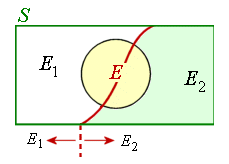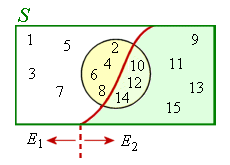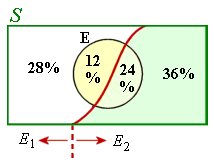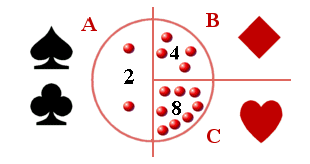Search IntMath
Close

450+ Math Lessons written by Math Professors and Teachers

5 Million+ Students Helped Each Year

1200+ Articles Written by Math Educators and Enthusiasts

Simplifying and Teaching Math for Over 23 Years

# 10. Bayes' Theorem

Let E1 and E2 be two mutually exclusive events forming a partition of the sample space S and let E be any event of the sample space such that P(E) ≠ 0.### Example 1

The sample space S is described as "the integers 1 to 15" and is partitioned into:

E1 = "the integers 1 to 8" and

E2 = "the integers 9 to 15".

If E is the event "even number" then we have the following:[Recall from Conditional Probability that the notation P(E1 | E) means "the probability of the event E1 given that E has already occurred".]

## Statement of Bayes' Theorem

The probabilities for the situation described above is given by Bayes' Theorem, which can be calculated in two ways:

### Method 1

P(E_1 | E)=(P(E_1nnnE))/(P(E_1nnnE)+P(E_2nnnE))

=(P(E_1)xxP(E|E_1))/(P(E_1)xxP(E|E_1)+P(E_2)xxP(E|E_2)

So for our example above, checking both items of this equation:

P(E_1|E)=(P(E_1nnnE))/(P(E_1nnnE)+P(E_2nnnE))

=(4/15)/(4/15+3/15)

=4/7

### Method 2

We get the same result using the second form:

P(E_1|E)=(P(E_1)xxP(E|E_1))/(P(E_1)xxP(E|E_1)+P(E_2)xxP(E|E_2))

=(8/15xx4/8)/(8/15xx4/8+7/15xx3/7)

=(4/15)/(7/15)

=4/7

Continues below

## Extending Bayes' Theorem for Mutually Exclusive Events

Bayes' Theorem can be extended as follows:

If E1, E2, ... , Ek are mutually exclusive events forming partitions of the sample space S and if E is any event of S such that P(E) ≠ 0, then

P(E_i|E)=(P(E_i nnnE))/(P(E_1nnnE)+P(E_2nnnE)+...+P(E_knnnE))

### Example 2

Of all the smokers in a particular district, 40% prefer brand A and 60% prefer brand B. Of those smokers who prefer brand A, 30% are females, and of those who prefer brand B, 40% are female. What is the probability that a randomly selected smoker prefers brand A, given that the person selected is a female?S = "smokers in the district",

E1 = "prefer brand A",

E2 = "prefer brand B" and

E is the event "female".

P(E_1|E)=(P(E_1nnnE))/(P(E_1nnnE)+P(E_2nnnE))

=(12/100)/(12/100+24/100)

=1/3

Or equivalently:

P(E_1|E) = (P(E_1)xxP(E|E_1))/(P(E_1)xxP(E|E_1)+P(E_2)xxP(E|E_2))

=(0.4xx0.3)/(0.4xx0.3+0.6xx0.4)

=1/3

### Example 3

There are 3 urns A, B and C each containing a total of 10 marbles of which 2, 4 and 8 respectively are red. A pack of cards is cut and a marble is taken from one of the urns depending on the suit shown - a black suit indicating urn A, a diamond urn B, and a heart urn C. What is the probability a red marble is drawn?

If somebody secretly cut the cards and drew out a marble and then announced to us a red marble had in fact been drawn, could we compute the probability of the cut being, say, a heart (or more generally, can we compute the probability of a specified prior event given that the subsequent event did occur)?P(R)=P(A)xxP(R|A)+ P(B)xxP(R|B)+ P(C)xxP(R|C)

=26/52xx2/10+ 13/52xx4/10+ 13/52xx8/10

=2/5

P(H|R) =(P(H)xxP(R|H))/(P(R)) =(13/52xx8/10)/(4/10) =1/2

P(D|R) =(P(D)xxP(R|D))/(P(R)) =(13/52xx4/10)/(4/10) =1/4

P(Bl|R) =(P(Bl)xxP(R|Bl))/(P(R)) =(26/52xx2/10)/(4/10) =1/4

Conclusion: The probabilities add to 1, so we can compute the probability of a specified event given the subsequent event did occur.

## Further explanation of Bayes' Theorem

Here's 2 video explanations of Bayes' Theorem:

Friday math movie – NUMB3RS and Bayes’ Theorem

## Problem SolverThis tool combines the power of mathematical computation engine that excels at solving mathematical formulas with the power of GPT large language models to parse and generate natural language. This creates math problem solver thats more accurate than ChatGPT, more flexible than a calculator, and faster answers than a human tutor. Learn More.